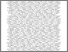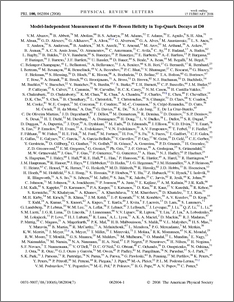# Model-independent measurement of the W boson helicity in top quark decays

Collaboration, D0 and M. Abazov, V. and Bertram, Iain and Borissov, Guennadi and Fox, Harald and Love, Peter and Rakitin, Alexander and Ratoff, Peter and Sopczak, Andre and Williams, Mark (2008) Model-independent measurement of the W boson helicity in top quark decays. Physical review letters, 100 (6). ISSN 1079-7114Preview
PDF
paper_090.pdf - Published Version

We present the first model-independent measurement of the helicity of $W$ bosons produced in top quark decays, based on a 1 fb$^{-1}$ sample of candidate $t\bar{t}$ events in the dilepton and lepton plus jets channels collected by the D0 detector at the Fermilab Tevatron $p\bar{p}$ Collider. We reconstruct the angle $\theta^*$ between the momenta of the down-type fermion and the top quark in the $W$ boson rest frame for each top quark decay. A fit of the resulting \costheta distribution finds that the fraction of longitudinal $W$ bosons $f_0 = 0.425 \pm 0.166 \hbox{(stat.)} \pm 0.102 \hbox{(syst.)}$ and the fraction of right-handed $W$ bosons $f_+ = 0.119 \pm 0.090 \hbox{(stat.)} \pm 0.053 \hbox{(syst.)}$, which is consistent at the 30% C.L. with the standard model.View Item